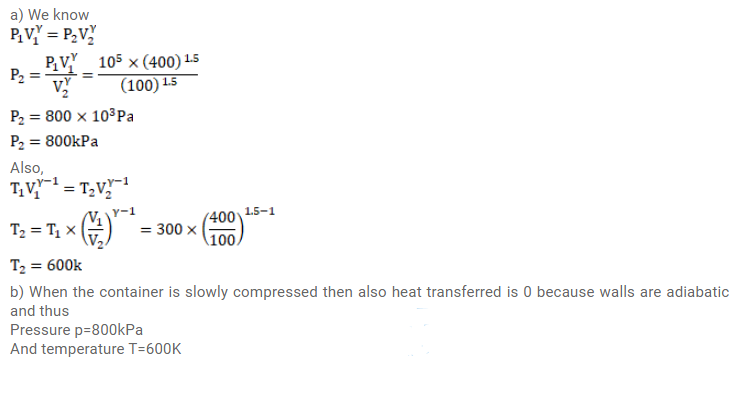# A gas is enclosed in a cylindrical can fitted with a piston.Question:

A gas is enclosed in a cylindrical can fitted with a piston. The walls of the can and the piston are adiabatic. The initial pressure, volume and temperature of the gas are $100 \mathrm{kPa}, 400 \mathrm{~cm}^{3}$ and $300 \mathrm{~K}$ respectively. The ratio of the specific heat capacities of the gas is $C_{p} / C_{v}=1.5$.

Find the pressure and the temperature of the gas if it is (a) suddenly compressed (b) slowly compressed to $100 \mathrm{~cm}^{3}$.

Solution: